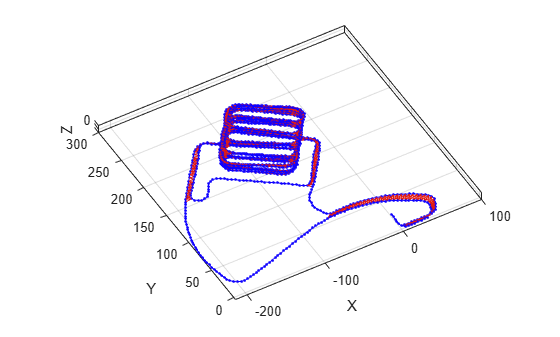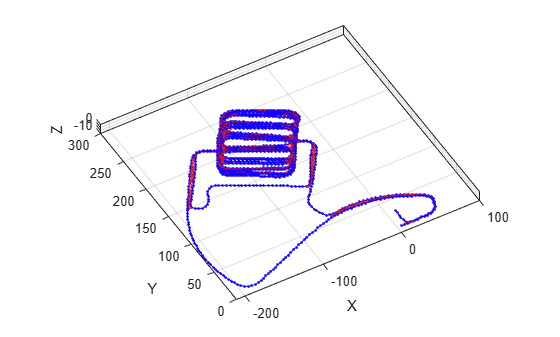# optimizePoseGraph

Optimize nodes in pose graph

## Syntax

``updatedGraph = optimizePoseGraph(poseGraph)``
``updatedGraph = optimizePoseGraph(poseGraph,solver)``
``[updatedGraph,solutionInfo] = optimizePoseGraph(___)``
``[___] = optimizePoseGraph(___,Name,Value)``

## Description

example

````updatedGraph = optimizePoseGraph(poseGraph)` adjusts the poses based on their edge constraints defined in the specified graph to improve the overall graph. You optimize either a 2-D or 3-D pose graph. The returned pose graph has the same topology with updated nodes. ```
````updatedGraph = optimizePoseGraph(poseGraph,solver)`specifies the solver type for optimizing the pose graph.```
````[updatedGraph,solutionInfo] = optimizePoseGraph(___)` returns additional statistics about the optimization process in `solutionInfo` using any of the previous syntaxes.```
````[___] = optimizePoseGraph(___,Name,Value)` specifies additional options using one or more `Name,Value` pairs. For example, `'MaxIterations',1000` increases the maximum number of iterations to 1000.```

## Examples

collapse all

Optimize a pose graph based on the nodes and edge constraints. The pose graph used in this example is taken from the MIT Dataset and was generated using information extracted from a parking garage.

Load the pose graph from the MIT dataset. Inspect the `poseGraph3D` object to view the number of nodes and loop closures.

```load parking-garage-posegraph.mat pg disp(pg);```
``` poseGraph3D with properties: NumNodes: 1661 NumEdges: 6275 NumLoopClosureEdges: 4615 LoopClosureEdgeIDs: [1x4615 double] ```

Plot the pose graph with IDs off. Red lines indicate loop closures identified in the dataset.

```title('Original Pose Graph') show(pg,'IDs','off'); view(-30,45)```Optimize the pose graph. Nodes are adjusted based on the edge constraints and loop closures. Plot the optimized pose graph to see the adjustment of the nodes with loop closures.

```updatedPG = optimizePoseGraph(pg); figure title('Updated Pose Graph') show(updatedPG,'IDs','off'); view(-30,45)```## Input Arguments

collapse all

2-D or 3-D pose graph, specified as a `poseGraph` or `poseGraph3D` object.

Pose graph solver, specified as either `"builtin-trust-region"` or `"g2o-levenberg-marquardt"`. To tune either solver, use the name-value pair arguments for that solver.

### Name-Value Pair Arguments

Specify optional comma-separated pairs of `Name,Value` arguments. `Name` is the argument name and `Value` is the corresponding value. `Name` must appear inside quotes. You can specify several name and value pair arguments in any order as `Name1,Value1,...,NameN,ValueN`.

Example: `'MaxTime', 300`

### Note

Depending on the `solver` input, the function supports different name-value pairs.

#### If the `solver` input is set to `"builtin-trust-region"`:

collapse all

Maximum time allowed, specified as the comma-separated pair consisting of `'MaxTime'` and a positive numeric scalar in seconds. The optimizer exits after it exceeds this time.

Lower bound on the norm of the gradient, specified as the comma-separated pair consisting of `'GradientTolerance'` and a scalar. The norm of the gradient is calculated based on the cost function of the optimization. If the norm falls below this value, the optimizer exits.

Lower bound on the change in the cost function, specified as the comma-separated pair consisting of `'FunctionTolerance'` and a scalar. If the cost function change falls below this value between optimization steps, the optimizer exits.

Lower bound on the step size, specified as the comma-separated pair consisting of `'StepTolerance'` and a scalar. If the norm of the optimization step falls below this value, the optimizer exits.

Initial trust region radius, specified as a scalar.

Display intermediate iteration information on the MATLAB® command line, specified as the comma-separated pair consisting of `'VerboseOutput'` and either `'off'` or `'on'`.

IDs of loop closure edges in `poseGraph`, specified as the comma-separated pair consisting of `'LoopClosuresToIgnore'` and a vector. To get edge IDs from the pose graph, use `findEdgeID`.

Pose of the first node in `poseGraph`, specified as the comma-separated pair consisting of `'FirstNodePose'` and a pose vector.

For `poseGraph` (2-D), the pose is an ```[x y theta]``` vector, which defines the relative xy-position and orientation angle, `theta`.

For `poseGraph3D`, the pose is an ```[x y z qw qx qy qz]``` vector, which defines the relative xyz-position and quaternion orientation, `[qw qx qy qz]`.

### Note

Many other sources for 3-D pose graphs, including `.g2o` formats, specify the quaternion orientation in a different order, for example, ```[qx qy qz qw]```. Check the source of your pose graph data before adding nodes to your `poseGraph3D` object.

#### If the `solver` input is set to `"g2o-levenberg-marquardt"`:

collapse all

Maximum number of iterations, specified as the comma-separated pair consisting of `'MaxIterations'` and a positive integer. The optimizer exits after it exceeds this number of iterations.

Maximum time allowed, specified as the comma-separated pair consisting of `'MaxTime'` and a positive numeric scalar in seconds. The optimizer exits after it exceeds this time.

Lower bound on the change in the cost function, specified as the comma-separated pair consisting of `'FunctionTolerance'` and a scalar. If the cost function change falls below this value between optimization steps, the optimizer exits.

Display intermediate iteration information on the MATLAB command line, specified as the comma-separated pair consisting of `'VerboseOutput'` and either `'off'` or `'on'`.

IDs of loop closure edges in `poseGraph`, specified as the comma-separated pair consisting of `'LoopClosuresToIgnore'` and a vector. To get edge IDs from the pose graph, use `findEdgeID`.

Pose of the first node in `poseGraph`, specified as the comma-separated pair consisting of `'FirstNodePose'` and a pose vector.

For `poseGraph` (2-D), the pose is an ```[x y theta]``` vector, which defines the relative xy-position and orientation angle, `theta`.

For `poseGraph3D`, the pose is an ```[x y z qw qx qy qz]``` vector, which defines the relative xyz-position and quaternion orientation, `[qw qx qy qz]`.

### Note

Many other sources for 3-D pose graphs, including `.g2o` formats, specify the quaternion orientation in a different order, for example, ```[qx qy qz qw]```. Check the source of your pose graph data before adding nodes to your `poseGraph3D` object.

## Output Arguments

collapse all

Optimized 2-D or 3-D pose graph, returned as a `poseGraph` or `poseGraph3D` object.

Statistics of optimization process, returned as a structure with these fields:

• `Iterations` — Number of iterations used in optimization.

• `ResidualError` — Value of cost function when optimizer exits.

• `Exit Flag` — Exit condition for optimizer:

• `1` — Local minimum found.

• `2` — Maximum number of iterations reached. See `MaxIterations` name-value pair argument.

• `3` — Algorithm timed out during operation.

• `4` — Minimum step size. The step size is below the `StepTolerance` name-value pair argument.

• `5` — The change in error is below the minimum.

• `8` — Trust region radius is below the minimum set in `InitialTrustRegionRadius`.

 Grisetti, G., R. Kummerle, C. Stachniss, and W. Burgard. "A Tutorial on Graph-Based SLAM." IEEE Intelligent Transportation Systems Magazine. Vol. 2, No. 4, 2010, pp. 31–43. doi:10.1109/mits.2010.939925.

 Carlone, Luca, Roberto Tron, Kostas Daniilidis, and Frank Dellaert. "Initialization Techniques for 3D SLAM: a Survey on Rotation Estimation and its Use in Pose Graph Optimization." 2015 IEEE International Conference on Robotics and Automation (ICRA). 2015, pp. 4597–4604.